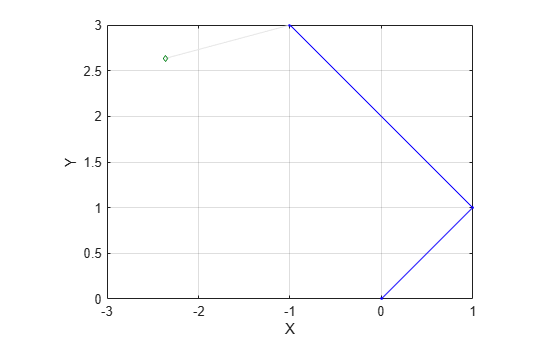# nodeEstimates

Poses of nodes in pose graph

Since R2019b

## Syntax

``measurements = nodeEstimates(poseGraph)``
``measurements = nodeEstimates(poseGraph,nodeIDs)``

## Description

example

````measurements = nodeEstimates(poseGraph)` lists all poses in the specified pose graph.```
````measurements = nodeEstimates(poseGraph,nodeIDs)` lists the poses with the specified node IDs.```

## Examples

collapse all

Create a 2-D pose graph object.

`pg = poseGraph;`

Add relative poses to the pose graph.

```addRelativePose(pg,[1 1 pi/2]); addRelativePose(pg,[2 2 pi/3]);```

Add a landmark point node to the last pose node in the pose graph.

```addPointLandmark(pg,[1 1]); show(pg);```List all poses in the pose graph.

`nodeEstimates(pg)`
```ans = 4×3 0 0 0 1.0000 1.0000 1.5708 -1.0000 3.0000 2.6180 -2.3660 2.6340 NaN ```

## Input Arguments

collapse all

Pose graph, specified as a `poseGraph` or `poseGraph3D` object.

Node IDs, specified as a positive integer or vector of positive integers. Each node added gets an ID sequentially in the graph.

## Output Arguments

collapse all

Measurements between nodes, returned as an n-by-3 matrix or n-by-7 matrix.

For `poseGraph` (2-D), each row is an `[x y theta]` vector, which defines the relative xy-position and orientation angle, `theta`, of a pose in the graph. For landmark positions, `theta` is returned as `NaN`.

For `poseGraph3D`, each row is an ```[x y z qw qx qy qz]``` vector, which defines the relative xyz-position and quaternion orientation, ```[qw qx qy qz]```, of a pose in the graph.

Note

Many other sources for 3-D pose graphs, including `.g2o` formats, specify the quaternion orientation in a different order, for example, `[qx qy qz qw]`. Check the source of your pose graph data before adding nodes to your `poseGraph3D` object.

## Version History

Introduced in R2019b

expand all